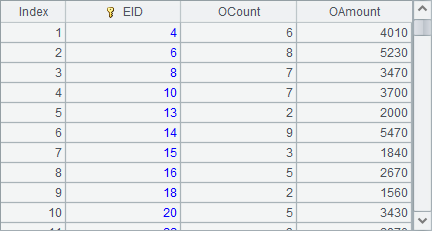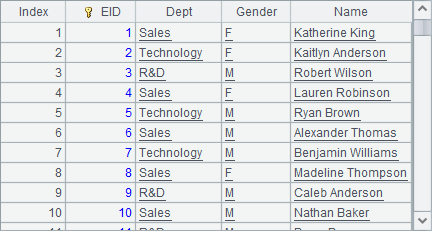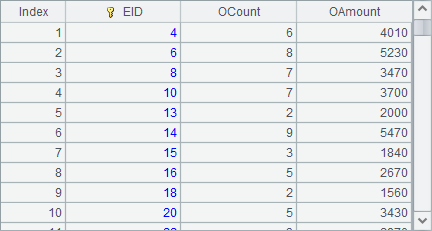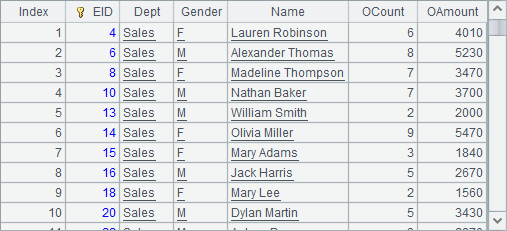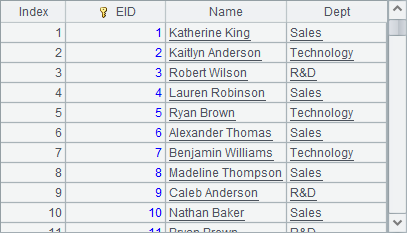# 轴图元

## 轴图元的轴线属性

 A 1 =canvas() 2 =demo.query("select * from GYMSCORE order by NAME") 3 =A1.plot("BackGround") 4 =A1.plot("EnumAxis","name":"x","axisColor":-16737895,"axisLineWeight":2,"axisArrow":256) 5 =A1.plot("NumericAxis","name":"y","location":2) 6 =A1.plot("Column","axis1":"x","data1":A2.(EVENT+","+NAME),"axis2":"y","data2":A2.(SCORE)) 7 =A1.plot("Legend","legendText":A2.id(NAME),"x":0.81,"y":0.2,"width": 0.18) 8 =A1.draw@p(450,250)

A2中获得绘图数据如下：## 轴图元的轴标题属性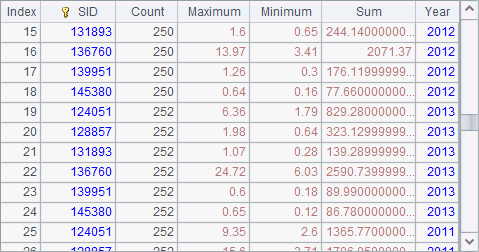## 轴图元的标签属性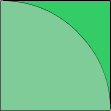## 轴图元的刻度线属性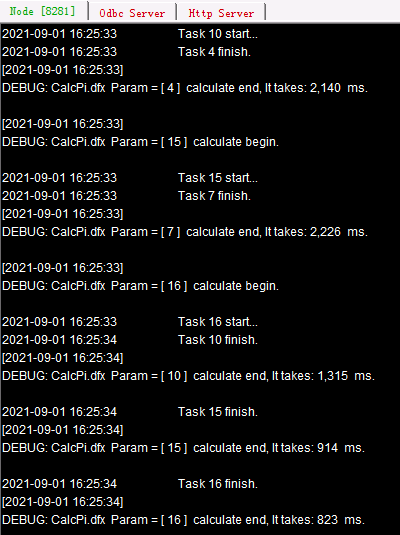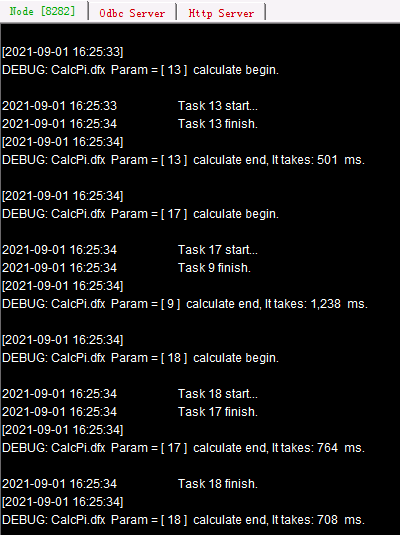## 轴图元的间隔区属性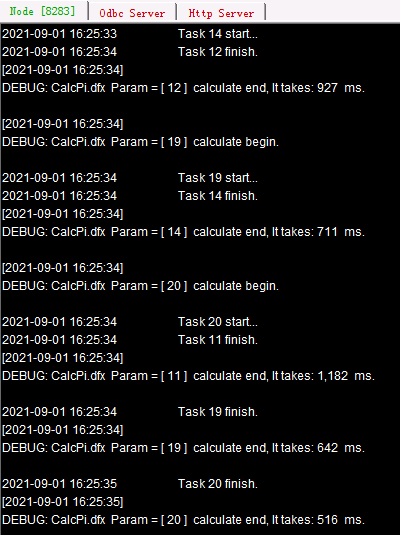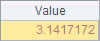## 极坐标中使用间隔区

 A B 1 =canvas() 2 =demo.query("select * from GYMSCORE") 3 =A1.plot("BackGround") =A1.plot("NumericAxis","name":"x","location":3, "autoCalcValueRange":false,"maxValue":16,"minValue":13, "scaleNum":3,"polarLength":0.24) 4 =A1.plot("EnumAxis","name":"y","location":4,"gapRatio":-0.5,"labelOverlapping":true) =A1.plot("MapAxis","name":"colors","logicalData": A2.id(NAME),"physicalData":[-65536,-16711936,-16776961]) 5 for A2.group(NAME) =A1.plot("Line","endToHead":true,"closedArea":true, "transparent":0,"lineWeight":2,"lineColor":A5.NAME: "colors","axis1":"x","data1":A5.(SCORE),"axis2":"y", "data2":A5.(EVENT)) 6 =A1.plot("Legend","legendText": A2.id(NAME),"x":0.81,"width":0.18, "legendType":3,"legendLineWeight":2, "legendLineColor":A2.id(NAME):"colors") 7 =A1.draw@p(500,300)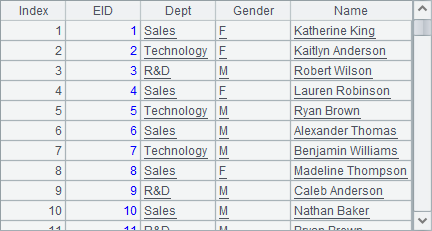A5中循环每位运动员的数据，并在B5中分别绘制折线图，其中折线的颜色使用到了刚刚的映射轴colors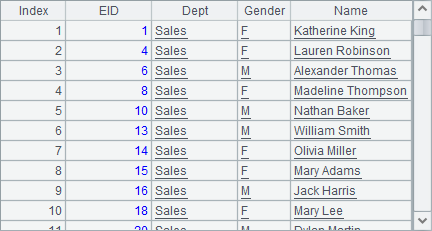A6中，绘制图例，同样使用了映射轴colors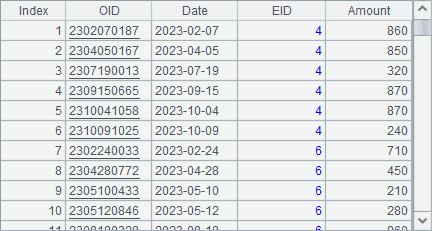A7中的绘图结果如下：